# Change in profit sharing ratio | Class 12

Change in profit sharing ratio occurs when there is change in either capital contribution of the partners or in active participation in the management. Sometimes it is decided by the existing partners to change their Profit sharing ratio. This change may result in gain to a few partners and loss to others. The proportion in which the partners acquires share is known as gaining ratio & the proportion in which the partners losses share is known as sacrificing ratio.

The formula for the same is:

Sacrificing Ratio = Old Ratio – New Ratio

Gaining Ratio = New Ratio – Old Ratio

## Question on Change in Profit Sharing Ratio are as given below:

Question 1 : -Change in Profit Sharing Ratio when assets or liabilities are revalued

A , B and C are partners sharing profits and losses equally.From 1st April 2016 they decide to share profits in the ratio of 6 : 3 : 1 .At the time of reconstitution,the following assets and liabilities are revalued and reassessed.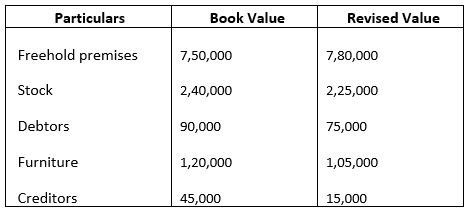Partners decided to record the above adjustments without affecting the book value of assets and liabilities The single entry to record adjustment will be:

Explanation : –

Net effect of revaluation:
Profit/Loss
Freehold premises = 30000
Stock = -15000
Debtors = -15000
Furniture = -15000
Creditors = 30000
= 15000
Profit on Revaluation 15000
Old profit sharing ratio = 1 : 1 : 1
New profit sharing ratio = 6 : 3 : 1
Sacrificing ratio = Old share – New share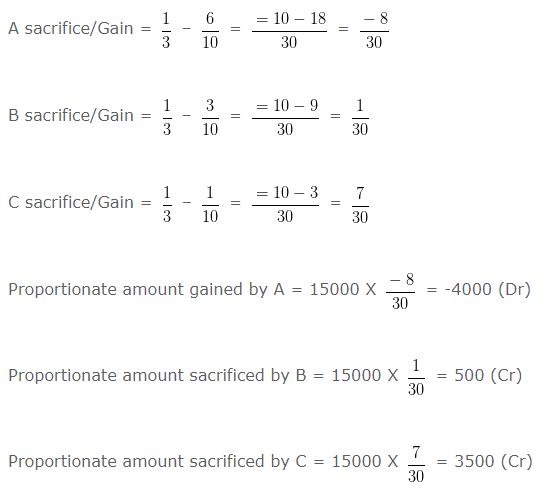A s capital A/c Dr 4000
To B s capital A/c 500
To C s capital A/c 500

### Change in Profit Sharing Ratio when goodwill adjusted

Question 2 : –

A , D and K are partners sharing profits and losses in the ratio of 1 : 3 : 1 respectively.From 1st April 2002 they decided to share the profits equally.For this purpose,the goodwill of the firm was valued at Rs. 150000 .The necessary journal enry for the treatment of goodwill on change in the profit sharing ratio will be:

Explanation : –

New profit sharing ratio = 1 : 1 : 1
Sacrificing ratio = Old share – New share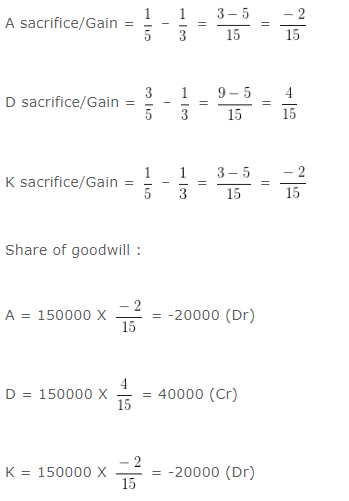A ‘s Capital Account Dr. 20000
K ‘s Capital Account Dr. 20000
To D ‘s Capital Account 40000

### Change in Profit Sharing Ratio when gaining partner acquires share

Question 3: –

A , D and K are partners sharing profits and losses in the ratio of 3 , 9 and 1 respectively. K acquires 2/3 share from D .New profit sharing ratio among partners will be:

Explanation : –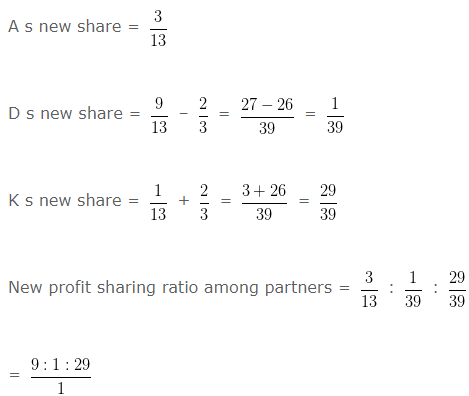= 9 : 1 : 29

### Change in Profit Sharing Ratio when partners sacrifice their share

Question 4 : –

B , C and D are partners sharing profits and losses in the ratio of 6 : 1 : 1 respectively. B sacrifices 1/2 of his share and C sacrifices 1/4th of his share in favour of D .What will be the new profit sharing ratio between partners.

Explanation : –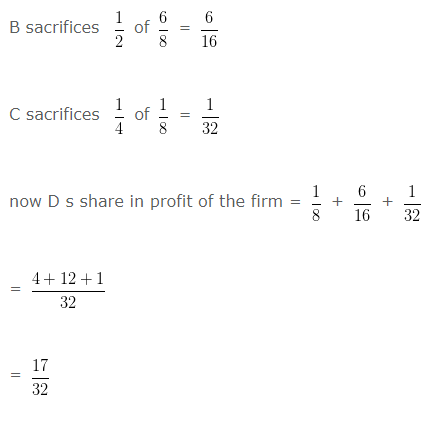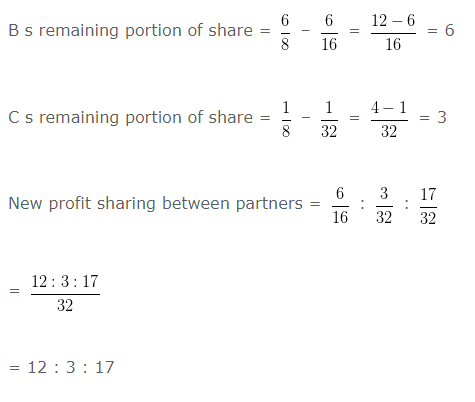### Change in Profit Sharing Ratio when Profit or Loss adjusted through Capital Account

Question 5 : –

A , B and C are partners sharing profits and losses in the ratio of 1 : 2 : 3 .They decide to share future profits in the ratio of 3 : 2 : 1 .They also decided to record the effect of the following without affecting their book values.
General reserve = 90000 Rs.
Profit and loss A/c(cr) = 30000 Rs.
The single adjusting entry will be:

Explanation : –

Calculation of net effect of reserves and accumulated profits/losses
General reserve = 90000 Rs.
(+) Profit and loss A/c(cr) = 30000 Rs.
Net effect = 60000 Rs.
Calculation of sacrificing/gaining share of partners:
Sacrificing ratio = Old share – New share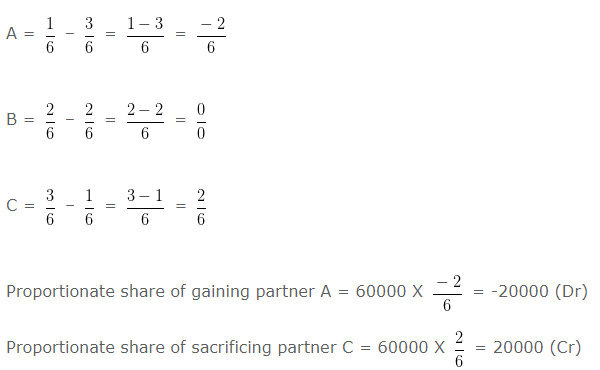To C s capital A/c 20000

### Change in Profit Sharing Ratio when Profit or Loss adjusted through Capital Account-II

Question 6 : –

A , D and K are partners sharing profits and losses in the ratio of 6 : 5 : 2 .They decide to share future profits in the ratio of 2 : 5 : 6 .They also decided to record the effect of the following without affecting their book values
Profit and loss A/c(cr) = 65000 Rs.
The single adjusting entry will be:

Explanation : –

Calculation of net effect of reserves and accumulated profits/losses
Profit and loss A/c(cr) = 65000 Rs.
Net effect = 52000 Rs.
Calculation of sacrificing/gaining share of partners
Sacrificing ratio = Old share – New share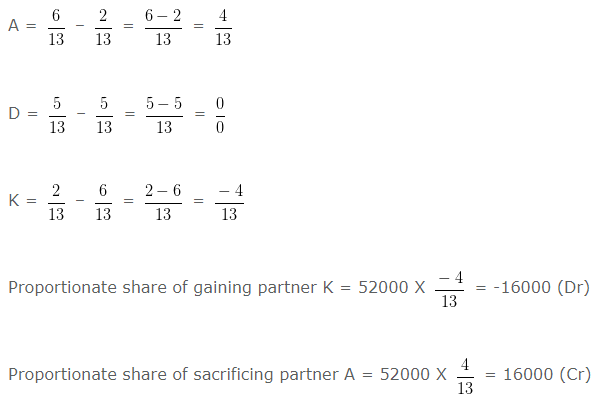K s capital A/c Dr 16000
To A s capital A/c 16000

### Change in Profit Sharing Ratio when partner acquires shares from 2 or more partners

Question 7 : –

A , D and K are partners sharing profits and losses in the ratio of 4 , 3 and 1 respectively. K acquires 1/5th share from A and D equally.New profit sharing ratio among partners will be:

Explanation : –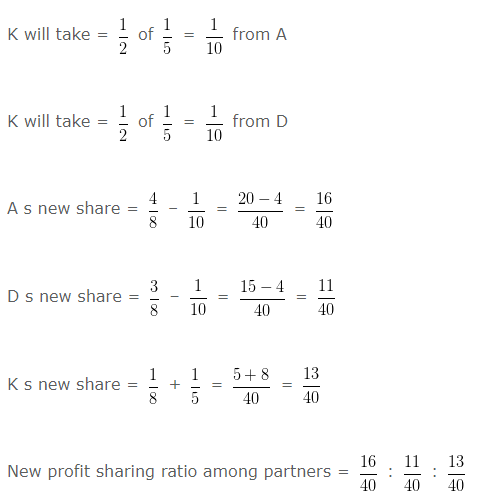= (16:11:13)/40

= 16 : 11 : 13

### Change in Profit Sharing Ratio when partners sacrifice their share

Question 8: –

A , D and K are partners sharing profits and losses in the ratio of 5 : 5 : 2 respectively. A sacrifices 1/4 of his share  and D sacrifices 1/4 of his share in favour of K .What will be the sacrificing ratio between partners.

Explanation : –

A sacrifices 1/4 of 5/12 = 5/48

D sacrifices 1/4 of 5/12 = 5/48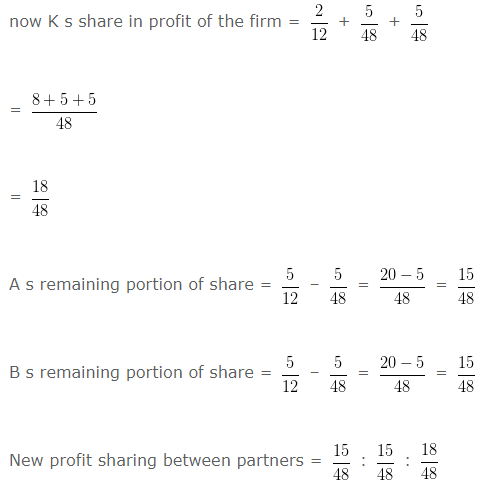= (15 : 15 : 18)/48

= 15 : 15 : 18

Sacrificing ratio = Old ratio – New ratio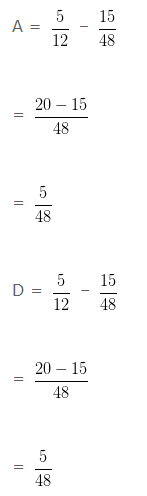5 : 5